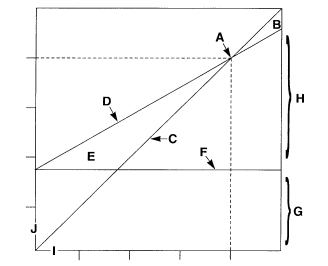/
/
/
21.Consider the Marshall Company’s segment analysis: Division A Division B Total Company Sales\$300,000\$200,000\$500,000 Va
Not my Question
Flag Content

# Question : 21.Consider the Marshall Company’s segment analysis: Division A Division B Total Company Sales\$300,000\$200,000\$500,000 Va : 1416285

21.Consider the Marshall Company’s segment analysis:

Division A    Division B    Total Company

Sales\$300,000\$200,000\$500,000

Variable costs150,000150,000300,000

Contribution margin150,00050,000200,000

Direct fixed costs50,00030,00080,000

Segment margin100,00020,000120,000

Allocated common fixed costs90,00060,000150,000

Operating income (loss)\$ 10,000\$(40,000)\$(30,000)

Common costs are allocated arbitrarily based on sales dollars.  If Marshall eliminates Segment B, what is the impact on the operating loss of the company?

a.The loss decreases by \$40,000.

b.The loss increases by \$20,000.

c.The loss decreases by \$60,000.

d.The loss increases by \$40,000.

22.A technique that uses the degrees of cost variability to measure the effect of changes in volume on resulting profits is:

a.Standard costing.

b.Variance analysis.

c.Cost-volume-profit analysis.

d.Segment profitability analysis.

23.Break-even sales volume in units is determined by:

a.Dividing the fixed cost by the difference between the unit selling price and unit variable costs.

b.Subtracting the fixed cost from the contribution margin.

c.Dividing the fixed cost by the unit selling price.

d.Subtracting the variable cost per unit from the unit selling price.

24.If the selling price and the variable cost per unit both increase 10 percent and fixed costs do not change, what is the effect on the contribution margin per unit and the contribution margin ratio?

a.Contribution margin per unit and the contribution margin ratio both remain unchanged.

b.Contribution margin per unit and the contribution margin ratio both increase.

c.Contribution margin per unit increases and the contribution margin ratio decreases.

d.Contribution margin per unit increases and the contribution ratio remains unchanged.

25.The  Company is planning to sell Product Z for \$10 a unit.  Variable costs are \$6 a unit and fixed costs are \$100,000.  What must total sales be to break even?

a.\$266,667

b.\$250,000

c.\$200,000

d.\$166,667

26.The Blue Saints Band is holding a concert in Toronto.  Fixed costs relating to staging a concert are \$350,000.  Variable costs per patron are \$5.00.  The selling price for a tickets \$25.00.  The Blue Saints Band has sold 23,000 tickets so far.

How many tickets does the Blue Saints Band need to sell to break even?

a.23,000

b.20,000

c.14,000

d.17,500

27.Consider the income statement for Pickbury Farm:

Sales\$500,000

Variable costs350,000

Contribution margin150,000

Fixed costs80,000

Net income\$ 70,000

What is the break-even point in sales dollars (rounded to the nearest dollar)?

a.\$714,286

b.\$500,000

c.\$266,667

d.\$120,000

28.Each of the following would affect the break-even point except a change in the:

a.Variable cost per unit.

b.Total fixed costs.

c.Sales price per unit.

d.Number of units sold.

29.Tennenholtz Company’s break-even graph is depicted below.  The line labeled “D” is:a.The sales line.

b.The contribution margin line.

c.The total cost line.

d.The variable cost line.

30.Tennenholtz Company’s break-even graph is depicted below.  Which area indicates the profitability of the company’s product?a.E.

b.G.

c.B.

d.H.

## Solution 5 (1 Ratings )

Solved
Accounting 3 Years Ago 441 Views